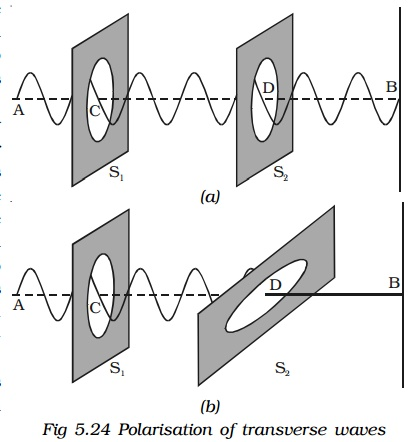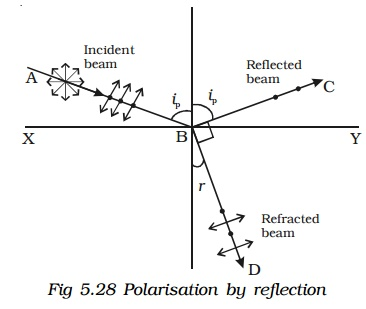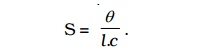Home | | Physics | Polarisation

# Polarisation

The phenomena of reflection, refraction, interference, diffraction are common to both transverse waves and longitudinal waves. But the transverse nature of light waves is demonstrated only by the phenomenon of polarisation.

Polarisation

The phenomena of reflection, refraction, interference, diffraction are common to both transverse waves and longitudinal waves. But the transverse nature of light waves is demonstrated only by the phenomenon of polarisation.

## 1. Polarisation of transverse waves.

Let a rope AB be passed through two parallel vertical slits S1 and S2 placed close to each other. The rope is fixed at the end B. If the free end A of the rope is moved up and down perpendicular to its length,transverse waves are generated with vibrations parallel to the slit. These waves pass through both S1 and S2 without any change in their amplitude. But if S2 is made horizontal, the two slits are perpendicular to each other. Now, no vibrations will pass through S2 and amplitude of vibrations will become zero. i.e the portion S2B is without wave motion as shown in fig 5.24.

On the otherhand, if longitudinal waves are generated in the rope by moving the rope along forward and backward, the vibrations will pass through S1 and S2 irrespective of their positions.

This implies that the orientation of the slits has no effect on the propagation of the longitudinal waves, but the propagation of the transverse waves, is affected if the slits are not parallel to each other.

A similar phenomenon has been observed in light, when light passes through a tourmaline crystal.Light from the source is allowed to fall on a tourmaline crystal which is cut parallel to its optic axis (Fig. 5.25a).

The emergent light will be slightly coloured due to natural colour of the crystal. When the crystal A is rotated, there is no change in the intensity of the emergent light. Place another crystal B parallel to A in the path of the light. When both the crystals are rotated together, so that their axes are parallel, the intensity of light coming out of B does not change. When the crystal B alone is rotated, the intensity of the emergent light from B gradually decreases. When the axis of B is at right angles to the axis of A, no light emerges from B (Fig. 5.25b).

If the crystal B is further rotated, the intensity of the light coming out of B gradually increases and is maximum again when their axis are parallel.

Comparing these observations with the mechanical analogue discussed earlier, it is concluded that the light waves are transverse in nature.

Light waves coming out of tourmaline crystal A have their vibrations in only one direction, perpendicular to the direction of propagation. These waves are said to be polarised. Since the vibrations are restricted to only one plane parallel to the axis of the crystal, the light is said to be plane polarised. The phenomenon of restricting the vibrations into a particular plane is known as polarisation.

## 2. Plane of vibration and plane of polarisation

The plane containing the optic axis in which the vibrations occur is known as plane of vibration. The plane which is at right angles to the plane of vibration and which contains the direction of propagation of the polarised light is known as the plane of polarisation. Plane of polarisation does not contain vibrations in it.In the Fig 5.26 PQRS represents the plane of vibration and EFGH represents the plane of polarisation.

## 3. Representation of light vibrations

In an unpolarised light, the vibrations in all directions may be supposed to be made up of two mutually perpendicular vibrations. These are represented by double arrows and dots (Fig 5.27).The vibrations in the plane of the paper are represented by double arrows, while the vibrations perpendicular to the plane of the paper are represented by dots.

## 4. Polariser and Analyser

A device which produces plane polarised light is called a polariser. A device which is used to examine, whether light is plane polarised or not is an analyser. A polariser can serve as an analyser and vice versa.

A ray of light is allowed to pass through an analyser. If the intensity of the emergent light does not vary, when the analyser is rotated, then the incident light is unpolarised; If the intensity of light varies between maximum and zero, when the analyser is rotated through 90o, then the incident light is plane polarised; If the intensity of light varies between maximum and minimum (not zero), when the analyser is rotated through 90o, then the incident light is partially plane polarised.

## 5. Polarisation by reflection

The simplest method of producing plane polarised light is by reflection. Malus, discovered that when a beam of ordinary light is reflected from the surface of transparent medium like glass or water, it gets polarised. The degree of polarisation varies with angle of incidence.

Consider a beam of unpolarised light AB, incident at any angle on the reflecting glass surface XY.Vibrations in AB which are parallel to the plane of the diagram are shown by arrows. The vibrations which are perpendicular to the plane of the diagram and parallel to the reflecting surface, shown by dots (Fig. 5.28).

A part of the light is reflected along BC, and the rest is refracted along BD. On examining the reflected beam with an analyser, it is found that the ray is partially plane polarised.

When the light is allowed to be incident at a particular angle, (for glass it is 57.5o) the reflected beam is completely plane polarised. The angle of incidence at which the reflected beam is completely plane polarised is called the polarising angle (ip).

## 6. Brewster’s law

Sir David Brewster conducted a series of experiments with different reflectors and found a simple relation between the angle of polarisation and the refractive index of the medium. It has been observed experimentally that the reflected and refracted rays are at right angles to each other, when the light is incident at polarising angle. From FigThe tangent of the polarising angle is numerically equal to the refractive index of the medium.

## 7. Pile of plates

The phenomenon of polarisation by reflection is used in the construction of pile of plates. It consists of a number of glass plates placed one over the other as shown in Fig 5.29 in a tube of suitable size. The plates are inclined at an angle of 32.5o to the axis of the tube. A beam of monochromatic light is allowed to fall on the pile of plates along the axis of the tube. So, the angle of incidence will be 57.5o which is the polarising angle for glass.The vibrations perpendicular to the plane of incidence are reflected at each surface and those parallel to it are transmitted. The larger the number of surfaces, the greater is the intensity of the reflected plane polarised light. The pile of plates is used as a polariser and an analyser.

## 8. Double refraction

Bartholinus discovered that when a ray of unpolarised light is incident on a calcite crystal, two refracted rays are produced. This phenomenon is called double refraction (Fig. 5.30a). Hence, two images of a single object are formed. This phenomenon is exhibited by several other crystals like quartz, mica etc.When an ink dot on a sheet of paper is viewed through a calcite crystal, two images will be seen (Fig 5.30b). On rotating the crystal, one image remains stationary, while the other rotates around the first. The stationary image is known as the ordinary image (O), produced by the refracted rays which obey the laws of refraction. These rays are known as ordinary rays. The other image is extraordinary image (E), produced by the refracted rays which do not obey the laws of refraction. These rays are known as extraordinary rays.

Inside a double refracting crystal the ordinary ray travels with same velocity in all directions and the extra ordinary ray travels with different velocities along different directions.

A point source inside a refracting crystal produces spherical wavefront corresponding to ordinary ray and elliptical wavefront corresponding to extraordinary ray.

Inside the crystal there is a particular direction in which both the rays travel with same velocity. This direction is called optic axis. The refractive index is same for both rays and there is no double refraction along this direction.

## 9. Types of crystals

Crystals like calcite, quartz, ice and tourmaline having only one optic axis are called uniaxial crystals.

Crystals like mica, topaz, selenite and aragonite having two optic axes are called biaxial crystals.

## 10. Nicol prism

Nicol prism was designed by William Nicol. One of the most common forms of the Nicol prism is made by taking a calcite crystal whose length is three times its breadth. It is cut into two halves along the diagonal so that their face angles are 720 and 1080. And the two halves are joined together by a layer of Canada balsam, a transparent cement as shown in Fig 5.31. For sodium light, the refractive index for ordinary light is 1.658 and for extraordinary light is 1.486. The refractive index for Canada balsam is 1.550 for both rays, hence Canada balsam does not polarise light.

A monochromatic beam of unpolarised light is incident on the face of the nicol prism. It splits up into two rays as ordinary ray (O) and extraordinary ray (E) inside the nicol prism (i.e) double refraction takes place. The ordinary ray is totally internally reflected at the layer of Canada balsam and is prevented from emerging from the other face. The extraordinary ray alone is transmitted through the crystal which is plane polarised. The nicol prism serves as a polariser and also an analyser.## 11. Polaroids

A Polaroid is a material which polarises light. The phenomenon of selective absorption is made use of in the construction of polariods. There are different types of polaroids.

A Polaroid consists of micro crystals of herapathite (an iodosulphate of quinine). Each crystal is a doubly refracting medium, which absorbs the ordinary ray and transmits only the extra ordinary ray. The modern polaroid consists of a large number of ultra microscopic crystals of herapathite embedded with their optic axes, parallel, in a matrix of nitro –cellulose.

Recently, new types of polariod are prepared in which thin film of polyvinyl alcohol is used. These are colourless crystals which transmit more light, and give better polarisation.

## 12. Uses of Polaroid

1.     Polaroids are used in the laboratory to produce and analyse plane polarised light.

2.     Polaroids are widely used as polarising sun glasses.

3.     They are used to eliminate the head light glare in motor cars.

4.     They are used to improve colour contrasts in old oil paintings.

5.     Polaroid films are used to produce three – dimensional moving pictures.

6.     They are used as glass windows in trains and aeroplanes to control the intensity of light. In aeroplane one polaroid is fixed outside the window while the other is fitted inside which can be rotated. The intensity of light can be adjusted by rotating the inner polaroid.

7.     Aerial pictures may be taken from slightly different angles and when viewed through polaroids give a better perception of depth.

8.     In calculators and watches, letters and numbers are formed by liquid crystal display (LCD) through polarisation of light.

9.     Polarisation is also used to study size and shape of molecules.

## 13. Optical activity

When a plane polarised light is made to pass through certain substances, the plane of polarisation of the emergent light is not the same as that of incident light, but it has been rotated through some angle. This phenomenon is known as optical activity. The substances which rotate the plane of polarisation are said to be optically active. Examples : quartz, sugar crystals, turpentine oil, sodium chloride etc.

Optically active substances are of two types, (i) Dextrorotatory (right handed) which rotate the plane of polarisation in the clock wise direction on looking towards the source. (ii) Laevo – rotatory (left handed) which rotate the plane of polarisation in the anti clockwise direction on looking towards the source.

Light from a monochromatic source S, is made to pass through a polariser P. The plane polarised light is then made to fall on an analyser A, which is in crossed position with P. No light comes out of A. When a quartz plate is inserted between the polariser and analyser some light emerges out of the analyzer A (Fig. 5.32). The emerging light is cut off again, when the analyzer is rotated through a certain angle.

This implies that light emerging from quartz is still plane polarised, but its plane of polarisation has been rotated through certain angle.The amount of optical rotation depends on :

§  thickness of crystal

§  density of the crystal or concentration in the case of solutions.

§  wavelength of light used

§  the temperature of the solutions.

## 14. Specific rotation

The term specific rotation is used to compare the rotational effect of all optically active substances.

Specific rotation for a given wavelength of light at a given temperature is defined as the rotation produced by one-decimeter length of the liquid column containing 1 gram of the active material in 1cc of the solution.

If θ is the angle of rotation produced by l decimeter length of a solution of concentration C in gram per cc, then the specific rotation S at a given wavelength λ for a given temperature t is given byThe instrument used to determine the optical rotation produced by a substance is called polarimeter.

Sugar is the most common optically active substance and this optical activity is used for the estimation of its strength in a solution by measuring the rotation of plane of polarisation.

Study Material, Lecturing Notes, Assignment, Reference, Wiki description explanation, brief detail
Physics : Electromagnetic Waves and Wave optics : Higher Secondary(12 Std) : Polarisation |